# HBSE 6th Class Maths Solutions Chapter 3 Playing With Numbers Ex 3.7

Haryana State Board HBSE 6th Class Maths Solutions Chapter 3 Playing With Numbers Ex 3.7 Textbook Exercise Questions and Answers.

## Haryana Board 6th Class Maths Solutions Chapter 3 Playing With Numbers Exercise 3.7

Question 1.
Renu purchases two bags of fertiliser of weights 75 kg and 69 kg. Find the maximum value of weight which can measure the weight of fertiliser exact number of times.
Solution:
Maximum value of weight which can measure exact number of times will be the H.C.F. of 75 and 69.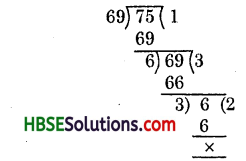∴ H.C.F. of 75 and 69 is 3:
Hence, maximum value of the required wt. = 3 kg

Question 2.
Three boys step off together from the same spot. Their steps measure 63 cm, 70 cm and 77 cm respectively. What is the minimum distance each should cover so that all can cover the distance in complete steps ?
Solution:
The required minimum distance each should walk must be a least common multiple of the measures of their steps.
Thus, we find the L.C.M. of 63, 70, 77.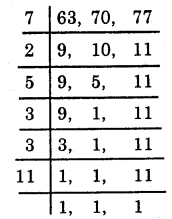∴ L.C.M. of 63, 70, 77 = 7 × 2 × 5 × 3 × 3 × 11 = 6930.
Hence, the required minimum distance is 6930 cm.Question 3.
The length, breadth and height of a room are 825 cm, 675 cm and 450 cm respectively. Find the longest tape which can measure the three dimensions of the room exactly.
Solution:
The longest tape which can measure the three dimensions of the room exactly will be the H.C.F. of 825, 675, 450.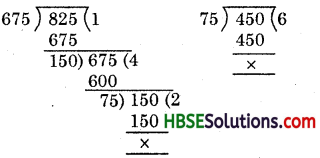∴ H.C.F. of 825, 675, 450 is 75.
Hence, the required longest tape will be 75 cm.

Question 4.
Determine the smallest 3-digit number which is exactly divisible by 6, 8 and 12.
Solution: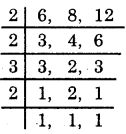∴ L.C.M. of 6, 8, 12= 2 × 2 × 3 × 2 = 24
Now, the smallest 3-digit number = 100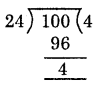24 – 4 = 20
Hence, the required number
= 100 + 20 = 120

Question 5.
Determine the largest 3-digit number exactly divisible by 8,10 and 12.
Solution: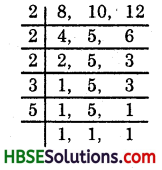∴ L.C.M. of 8, 10, 12
=2 × 2 × 2 × 3 × 5 = 120
Now, largest 3-digit number = 999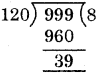Hence, the required number
= 999 – 39
= 960.Question 6.
The traffic lights at three different road crossings change after every 48 seconds, 72 seconds and 108 seconds respectively. If they change simultaneously at 7 A.M., at what time they will change simultaneously again?
Solution:
48 sec, 72 sec, 108 sec.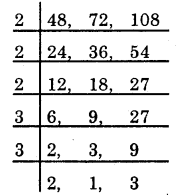∴ L.C.M. of 48, 72, 108
= 2 × 2 × 2 × 3 × 3 × 2 × 3
= 432 sec. = 7 min. 12 sec.
They will change simultaneously after 7 min. 12 sec
i.e., at 7 hr. 7 min. 12 sec. A.M.

Question 7.
Three tankers contain 403 litres, .434 litres and 465 litres of diesel respectively. Find the maximum capacity of a container that can measure the diesel of three containers exact number of times.
Solution:
The maximum capacity of a container will be the
H.C.F. of 403, 434, 465.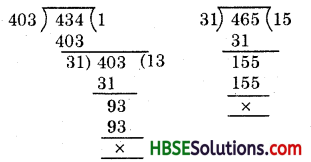∴ H.C.F. of 403, 434, 465 is 31.
Hence, maximum capacity of the required container = 31 litres. Ans.

Question 8.
Find the least number which when divided by 6, 15 and 18 leave remainder 5 in each case.
Solution:
We first find the L.C.M. of 6, 15 and 18 as follows :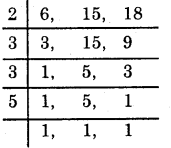∴ L.C.M. of 6, 15, 18 = 2 × 3 × 3 × 5 = 90
∴ 90 is the least number which when divided by the given numbers will leave remainder ‘0’ in each case. But we need the least number that leaves remainder 5 in each case.
Hence, the required number = 90 + 5 = 95.Question 9.
Find the smallest four-digit number which is divisible by 18,24 and 32.
Solution:
We first find the L.C.M. of 18, 24, 32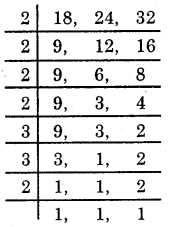∴ L.C.M. of 18, 24, 32 .
= 2 × 2 × 2 × 2 × 3 × 3 × 2
= 288
Now, the smallest 4-digit number = 1000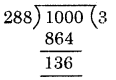288 – 136 = 152
Hence, the required number
= 1000 + 1.52= 1152Question 10.
Find the L.C.M. of the following numbers in which one number is always a multiple of 3 :
(a) 9 and 4
(b) 12 and 5
(c) 6 and. 5
(d) 15 and 4.
Observe a common property in the obtained L.C.M. Is L.C.M. the product of two numbers in each case ? Is L.C.M. always a multiple of 3 ?
Solution: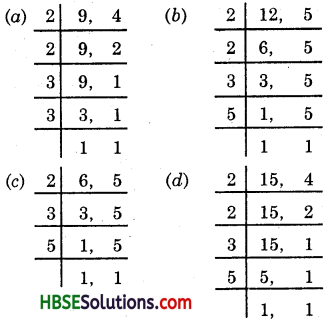(a) L.C.M.of 9 and 4 = 2 × 2 × 3 × 3 = 36
= 9 × 4
(b) L.C.M. of 12 and 5 = 2 × 2 × 3 × 5
60 = 12 × 5
(c) LC.M. of 6 and 5 = 2 × 3 × 5 = 30 = 6 × 5
(d) L.C.M.of 15 and 4 = 2 × 2 × 3 × 5 = 60 = 15 × 4
Yes, L.C.M. is equal to the product of two numbers in each case.
Yes, L.C.M. is always a multiple of 3.